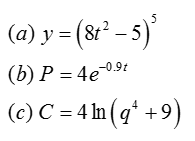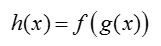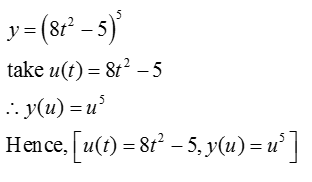# Use the variable u for the inside function to express each of the following as a composite function. Enclose arguments of functions in parentheses. For example, sin(2x). (a) y=(8t2−5)5 Enter your answer as a list with square brackets and terms separated by commas [u(t), y(u)], where u(t) is the inside function and y(u) is the outside function. (b) P=4e−0.9t Enter your answer as a list with square brackets and terms separated by commas [u(t), P(u)], where u(t) is the inside function and P(u) is the outside function.(c) C=4ln(q4+9) Enter your answer as a list with square brackets and terms separated by commas [u(q), C(u)], where u(q) is the inside function and C(u) is the outside function.

Question
74 views

Use the variable u for the inside function to express each of the following as a composite function.

Enclose arguments of functions in parentheses. For example, sin(2x).

(a) y=(8t2−5)5

Enter your answer as a list with square brackets and terms separated by commas [u(t), y(u)], where u(t) is the inside function and y(u) is the outside function.

(b) P=4e−0.9t

Enter your answer as a list with square brackets and terms separated by commas [u(t), P(u)], where u(t) is the inside function and P(u) is the outside function.

(c) C=4ln(q4+9)

Enter your answer as a list with square brackets and terms separated by commas [u(q), C(u)], where u(q) is the inside function and C(u) is the outside function.

check_circle

Step 1

Given: Use the variable u for the inside function to express each of the following as a composite function.Step 2

concept used:

A composite function is a function that depends on another function.

Let f (x) and g(x) are two functions. they produce a new function h(x)Step 3

(a)...

### Want to see the full answer?

See Solution

#### Want to see this answer and more?

Solutions are written by subject experts who are available 24/7. Questions are typically answered within 1 hour.*

See Solution
*Response times may vary by subject and question.
Tagged in